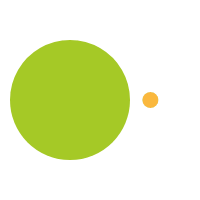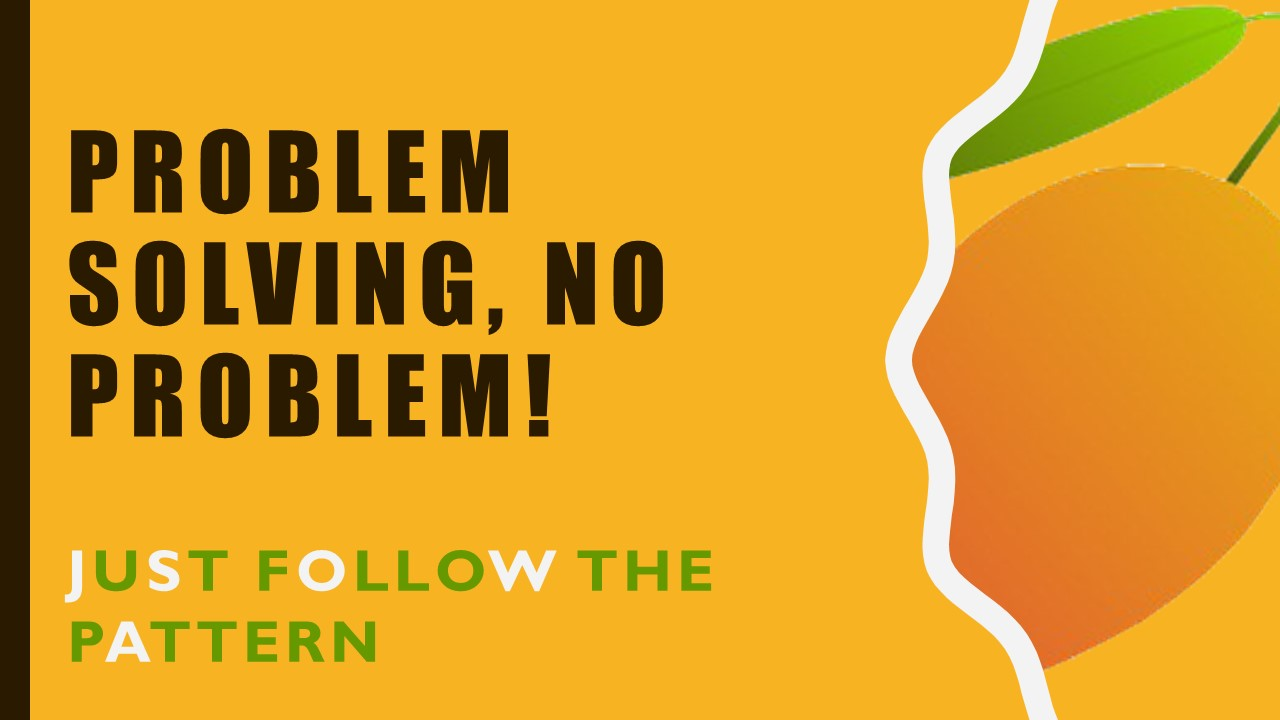July 14, 2018

# Problem Solving? “ No Problem “ Just follow the Pattern

I like puzzles because they tend to follow a pattern. I can see colors, shapes, number, which helps me to come to a solution. When I was in school I don't recall teachers or professors discussing patterns and looking for patterns to solve problems. Personally for me, I probably would have understood math a lot better if someone had taught me how to identify patterns in math.

According to a research paper in the Mathematics Education Research Journal by Joanne Mulligan & Michael Mitchelmore The power of mathematics lies in relations and transformations which give rise to patterns and generalizations. Abstracting patterns is the basis of structural knowledge, the goal of mathematics learning

## Starting Early with Repeating Patterns

Kindergarten students often work on finding a pattern.

They have images like this, which are called repeating patterns, following a rule like, ABAB or ABCABC or ABBC, ABBC. There are many variations and having students start to recognize these patterns is important. According to the above article, students that have good visualization skills, which involves recognition of pattern and structure, have increased mathematical achievement and analogical reasoning.

These repetitive pattern problems are great ways to get those young children to start the mental development of critical thinking and analytical sense. I have found that with young children when presented with numerous objects of color they will first notice the color, then shape and then number. This is helpful in upper grades when teachers work with students on algebraic equations.

A great game to work on this age level on pattern recognition is a game called BLINK. This game has students quickly noticing color, shape and number. It is a fun and easy game to play.

## Branch out into Growing Patterns

From repeating patterns students can move on to growing patterns.

I like using a number grid. This activity Number Neighbors and Number Neighbors II from our 1st and 2nd grade math kits seem simple but it can be challenging as you start to apply the numbers.

The students start with an unmarked grid, the center number can be a random number selected by the teacher, 44. Students are then to start filling in the blank squares like it is a section of the number grid. Some of the squares have circles in them, this is to help focus the students on filling out the easiest to solve numbers first (an example of how shapes can focus students visualization). What number comes directly before 44? What numbers comes directly after 44? What number would be directly above 44? How many less than 44 is that?

This grid has students developing the patterning of one more (+1), one less (-1), ten more (+10), ten less (-10) as they fill in the numbers.

## Patterns Keep on Growing

Another patterning game which I think is a great visualization activity is the game Jump Around. Having student starting on a certain number, 3. Now ask them to jump 5 and keep track of the numbers they land, 3, 8, 13, 18, 23â€¦ What pattern do the students notice, what will be the next number in the pattern? Can we determine what the 10 number in the pattern? Students may struggle with the circular pattern of this number line, if that is the case have them use a number grid. Color in the numbers as they land on them, so the patterning because more visual.

## Stick-With-It Patterns will Develop

Growing patterns can be shown visually using sticks, squares, pattern blocks. Stick To It has students determining a pattern using sticks. Matchstick problems are very common in middle school.

First square has 4 sticks, second square has 8, third square has 12. Can student determine the number of sticks in the 10th square or the 100th square? What pattern is being followed.

## Algebra and Patterns

The final pattern game I will show is developed from those math tricks you may have seen before. Tricks like these.

Choose a number

Multiply by 3

Divide by 3

Subtract 1st number

I can tell you what the answer is.

How will I always know, no matter what number you start with?

Use squares and circles to see how this works.

Square represents the number

Multiply by 3

Divide by 3

Subtract first number

Patterns can help students who find math difficult and uninspiring into understandable and visual. Helping them see how patterning can help them become better mathematicians.